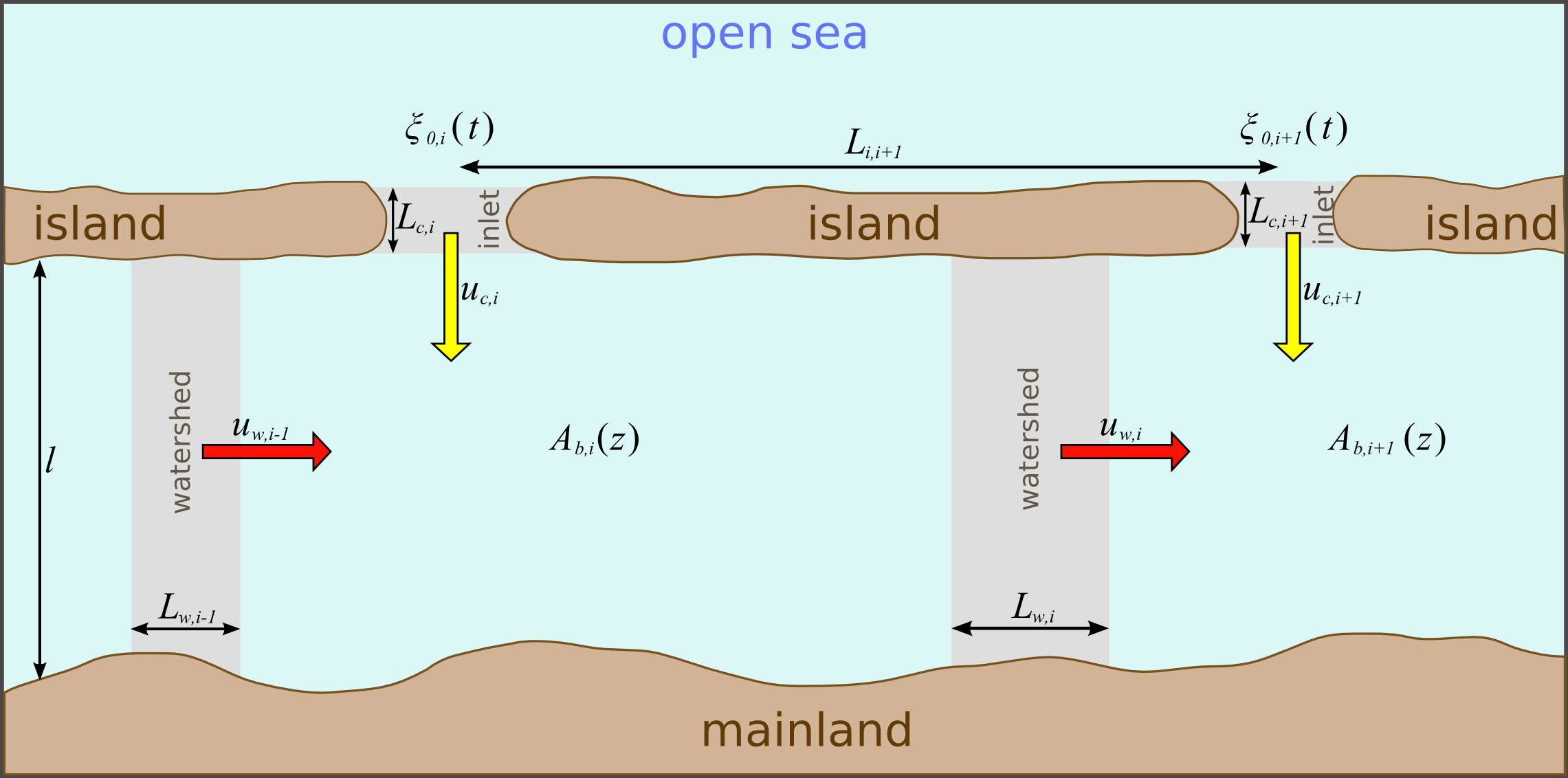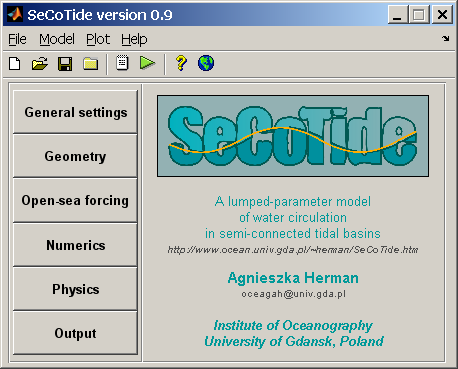#Model of Semi-Connected Tidal Inlets

Agnieszka Herman

Institute of Oceanography, University of Gdansk, Poland

SeCoTide is a lumped-parameter numerical model of water circulation in a system of tidal basins connected to the open sea by narrow inlets and to each other by shallow "transition areas" (tidal divides or watersheds), as shown schematically in this figure:The basins are treated as water reservoirs with volumes determined from their bathymetric curves. Both inlets and watersheds are parameterized as one-dimensional channels with a given length, cross-sectional area and water depth. For N basins, the model solves a system of 3N−1 ordinary differential equations (ODEs): N continuity equations for the volume change of each basin, N momentum equations for the inlets and N−1 momentum equations for the watersheds. The flow within the inlets and watersheds is assumed to be driven by water level differences at both ends and damped by friction.

The most important features of SeCoTide are:

• The model can be run for any number of basins N.
• The basin surface areas can be described by any nondecreasing function of z, Ab(z). Similarly, the cross-sectional areas and mean depths of the inlets and of the watersheds depend on water level: Ac(z), Aw(z) and hc(z), hw(z), respectively.
• The user can provide the input parameters for the model either in a dimensional form or in terms of parameters analogous e.g to the Helmholtz frequency defined traditionally for single tidal inlets (see the model description for details).
• Based on time series of sea-surface elevation at the entrance to each inlet the model calculates time series of sea levels in each basin and of the current velocities through the inlets and watersheds.
• The model equations are written in a matrix form and solved by means of variable-order multistep ODE solver available in MATLAB.
• SeCoTide can be run via a simple, easy-to-use Graphical User's Interface (GUI) with help in HTML describing all technical details of its usage. The computational part of the programme (MATLAB m-file) is available as well (see the Download section). This is how the main window of the present SeCoTide version 0.9 looks like:The model has been successfully applied to study various aspects of water circulation in the basins of the East Frisian Wadden Sea (Germany) and of the Magdalen Islands (Gulf of Saint-Lawrence, Canada) – see references. SeCoTide is conceptionally very similar to the model of two connected basins developed recently by van de Kreeke et al. (Coastal Engng 2008, 55, 319–332).

### References

Herman, A., 2007. Numerical modelling of water transport processes in partially-connected tidal basins. Coastal Engng, 54, 297-320, doi:10.1016/j.coastaleng.2006.10.003. (article)

Urbański, J.A., Herman, A., 2009. Water exchange between the basins of the German Wadden Sea studied with a coupled Matlab-ArcGIS model. J. Coastal Res., SI 56 (Proc. 10th Int. Coastal Symp., Lisbon, Portugal), 1085-1089. (article)